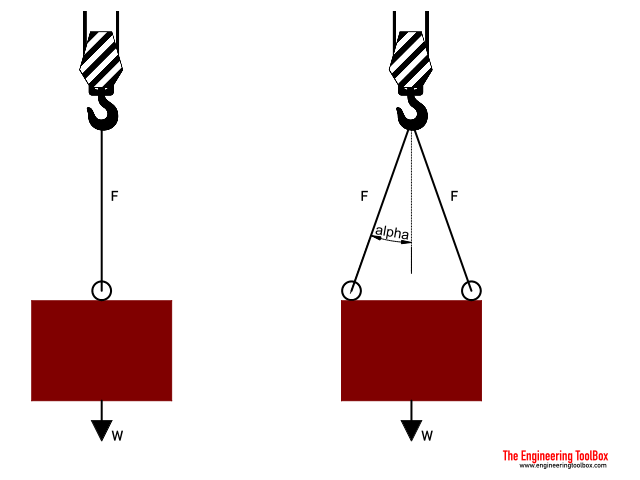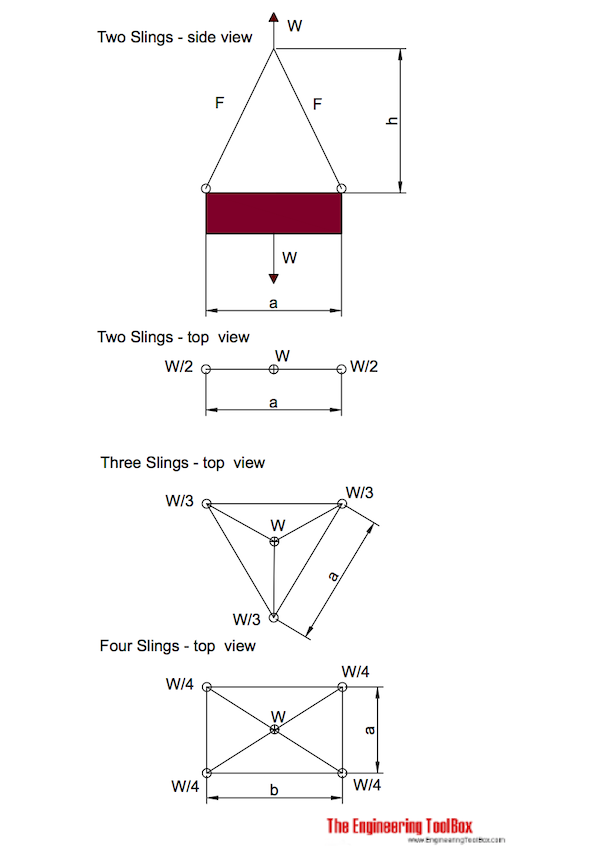Engineering ToolBox - Resources, Tools and Basic Information for Engineering and Design of Technical Applications!

# Wire Rope Slings

## Sling angles and influence on capacity.

Slings angles affects ropes capacities.If angle - alpha - is measured between

• the vertical line (as with gravity force), and
• the rope or wire

the relative capacity compared to a vertical straight lifting is reduced with reduction factor as indicated below.

f = cos(α)                           (1)

where

f = reduction factor

α = angle between vertical line and rope (degrees)

Angle
- α -
(degrees)
Reduction Factor
- f -
0 1.000
10 0.985
20 0.940
30 0.866
40 0.766
50 0.643
60 0.500
70 0.342

### Example - Capacity of a Single Rope or Wire

The capacity of a single rope that follows a vertical line is 100% since the reduction factor is 1.

If the weight of a body is W - the load in the wire is

F = W                                     (2)

where

F = force in rope (N, lbf)

W = m g = weight of body (N, lbf)

m = mass of body (kg, slugs)

g = acceleration of gravity (9.81 m/s2, 32.17 ft/s2)

For a body with mass 100 kg the load in the rope can be calculated

F = (100 kg) (9.81 m/s2)

= 981 N

= 9.8 kN

### Example - Capacity of Two Ropes (or Wires)

#### Two wires or ropes follows the vertical line

The capacity of two wires that follows the vertical line is 100% since the reduction factor is 1.

If the weight of a body is W - the load in each wire is

F = W / 2                                  (3)

For a body with weight 1000 N the load in each rope can be calculated as

F = (1000 N) / 2

= 500 N

= 0.5 kN

#### Two wires - or ropes - with angle 30o to the vertical line

The capacity of two wires with angle 30o to the vertical line is 86.6% since the reduction factor is 0.866.

If the weight of a body is W - the load in each wire is

F = (W / 2) / cos(30o)

= (W / 2) / f

= (W / 2) / 0.866

= 0.577 W                                          (4)

For a body with weight 1000 N the loads in the ropes can be calculated

F = 0.577 (1000 N)

= 577 N

= 0.58 kN

### Wire Rope Slings Calculators

The calculators below can be used to calculate wire rope forces. Note that mass (kg) and not weight (N) is used as input.mass (kg)

length - a (m)

height - h (m)

mass (kg)

length - a (m)

height - h (m)

mass (kg)

length - a (m)

length - b (m)

height - h (m)

## Related Topics

• Mechanics - Forces, acceleration, displacement, vectors, motion, momentum, energy of objects and more.
• Statics - Loads - forces and torque, beams and columns.

## Engineering ToolBox - SketchUp Extension - Online 3D modeling!

Add standard and customized parametric components - like flange beams, lumbers, piping, stairs and more - to your Sketchup model with the Engineering ToolBox - SketchUp Extension - enabled for use with the amazing, fun and free SketchUp Make and SketchUp Pro .Add the Engineering ToolBox extension to your SketchUp from the SketchUp Pro Sketchup Extension Warehouse!

Translate

## Privacy

We don't collect information from our users. Only emails and answers are saved in our archive. Cookies are only used in the browser to improve user experience.

Some of our calculators and applications let you save application data to your local computer. These applications will - due to browser restrictions - send data between your browser and our server. We don't save this data.

## Citation

• Engineering ToolBox, (2010). Wire Rope Slings . [online] Available at: https://www.engineeringtoolbox.com/wire-rope-slings-d_1613.html [Accessed Day Mo. Year].

Modify access date.

. .

#### Scientific Online Calculator3 30